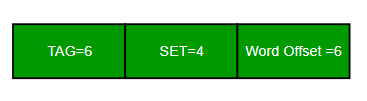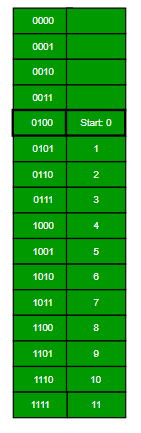Related Articles
GATE | GATE-CS-2007 | Question 81
• Last Updated : 19 Nov, 2018

Consider the data given in above question.

Which of the following lines of the data cache will be replaced by new blocks in accessing the array for the second time?
(A) line 4 to line 11
(B) line 4 to line 12
(C) line 0 to line 7
(D) line 0 to line 8

Explanation:

Size of Main Memory = 2^16 bytes
No. of lines = 32 = 2^5
Size of each line = 64 = 2^6 bytes => Word Offset = 6
2 way set is present
Hence, No.of sets = No. of lines / 2

= 2^5 / 2

= 2^4

Now, Main memory format= ////// Sir 1st image here ////////
Starting memory location : 1100 H => 0001 0001 0000 0000
Offset : 00 0000
Line   : 01 00 (4)This solution is contributed by Mohit Gupta.

My Personal Notes arrow_drop_up